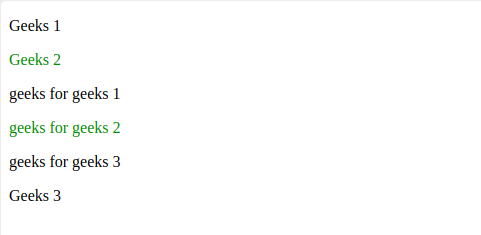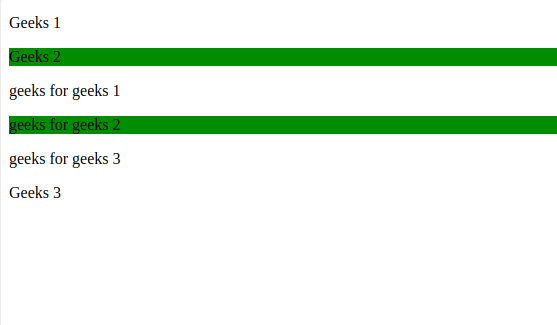GeeksforGeeks App
Open AppBrowser
Continue

# jQuery :nth-of-type() Selector

The :nth-of-type() is an inbuilt selector in jQuery, used to select all the nth-child elements of the specified parent.

Syntax:

` parent_name : nth-of-type(n|even|odd|algebraic equation)`

Parameters: It takes a parameter n | even | odd | algebraic equation.

Note:

• Child elements in different sections or divisions are treated differently
i.e, indexing starts from the beginning.
• In mn + c, n is starting from value 0.

Example 1: Using n as a parameter.

## HTML

 ```<``html``>``<``head``>``  ``<``script` `src``=``"https://ajax.googleapis.com/ajax/libs/jquery/3.3.1/jquery.min.js"``>``  ````  ``<``script``>``    ``\$(document).ready(function () {``      ``\$("p:nth-of-type(2)").css(``        ``"color", "green");``    ``});``  ``````<``body``>``  ``<``p``>Geeks 1``  ``<``p``>Geeks 2``  ``<``section``>``    ````    ``<``p``>geeks for geeks 1``    ``<``p``>geeks for geeks 2``    ``<``p``>geeks for geeks 3``  ````  ````  ``<``p``>Geeks 3`````

Output:In the above example, child element at index 2 (parent is p tag) formatted into green color i.e. “Geeks 2” and “geeks for geeks 2”.

Example 2: Using even as a parameter.

## HTML

 ```<``html``>``<``head``>``  ``<``script` `src``=``"https://ajax.googleapis.com/ajax/libs/jquery/3.3.1/jquery.min.js"``>``  ````  ``<``script``>``    ``\$(document).mouseover(function () {``      ``\$("p:nth-of-type(even)").css(``        ``"background-color", "green");``    ``});``  ``````<``body``>``  ``<``p``>Geeks 1``  ``<``p``>Geeks 2``  ``<``section``>``    ````    ``<``p``>geeks for geeks 1``    ``<``p``>geeks for geeks 2``    ``<``p``>geeks for geeks 3``  ````  ````  ``<``p``>Geeks 3`````

Output:In the above example, child element at even indices (parent is p tag) formatted into green color background i.e. “Geeks 2” and “geeks for geeks 2”.

Example 3: Using odd as a parameter.

## HTML

 ```<``html``>``<``head``>``  ``<``script` `src``=``"https://ajax.googleapis.com/ajax/libs/jquery/3.3.1/jquery.min.js"``>``  ````  ``<``script``>``    ``\$(document).mouseover(function () {``      ``\$("p:nth-of-type(odd)").css(``        ``"color", "red");``    ``});``  ``````<``body``>``  ``<``p``>Geeks 1``  ``<``p``>Geeks 2``  ``<``section``>``    ````    ``<``p``>geeks for geeks 1``    ``<``p``>geeks for geeks 2``    ``<``p``>geeks for geeks 3``  ````  ````  ``<``p``>Geeks 3`````

Output:In the above example, child element at odd indices (parent is p tag) formatted into red colour i.e. “Geeks 1”, “geeks for geeks 1”, “geeks for geeks 3” and “Geeks 3”.

Example 4: Using algebraic equation as a parameter.

## HTML

 ```<``html``>``<``head``>``  ``<``script` `src``=``"https://ajax.googleapis.com/ajax/libs/jquery/3.3.1/jquery.min.js"``>``  ````  ``<``script``>``    ``\$(document).mouseover(function () {``      ``\$("p:nth-of-type(3n+2)").css(``        ``"color", "green");``    ``});``  ``````<``body``>``  ``<``p``>Geeks 1``  ``<``p``>Geeks 2``  ``<``section``>``    ````    ``<``p``>geeks for geeks 1``    ``<``p``>geeks for geeks 2``    ``<``p``>geeks for geeks 3``    ``<``p``>geeks for geeks 4``    ``<``p``>geeks for geeks 5``  ````  ````  ``<``p``>Geeks 3`````

Output:In the above example, child element at indices value equal to 3n + 2 (parent is p tag) formatted into green colour i.e. “Geeks 2”, “geeks for geeks 2”, “geeks for geeks 5”.

My Personal Notes arrow_drop_up
Related Tutorials